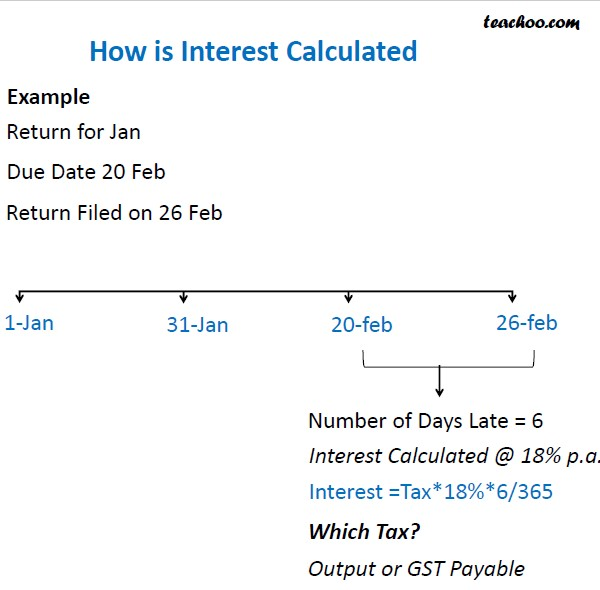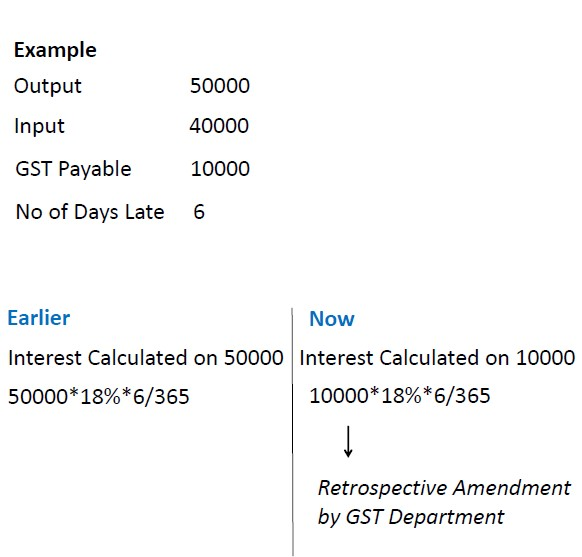Chapter 11 GST Interest and Late Fees Calculation

Courses
Free GST Certification Course

Interest is Calculated in GST as per following Formula

GST Payable*18%*No of Days/365

Let’s understand this with the help of an exampleQuestion

Is Interest Calculated in Case of GST Credit

Suppose Output is 30000, Input is 40000

What is Interest payable if tax paid 5 days late

Learn in your speed, with individual attention - Teachoo Maths 1-on-1 Class

### Transcript

How is Interest Calculated Return for Jan Due Date 20 Feb Return Filed on 26 Feb Number of Days Late = 6 1 Jan 31 Jan 20 feb 26 feb Interest Calculated @ 18% p.a. Interest =Tax*18%*6/365 Which Tax? Output or GST Payable Example Output Input GST Payable No of Days Late 50000 40000 10000 6 Earlier Interest Calculated on 50000 50000*18%*6/365 Now Interest Calculated on 10000 10000*18%*6/365 Retrospective Amendment by GST Department Example